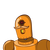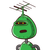# the angels of a quadrilateral are in the ratio 1:2:3:4. state the measure of its angles . answer​

the angels of a quadrilateral are in the ratio 1:2:3:4. state the measure of its angles . answer​

### 2 thoughts on “the angels of a quadrilateral are in the ratio 1:2:3:4. state the measure of its angles . answer​”

1.let the angles be

angle A = x°

angle B = 2x°

angle C = 3x°

angle D = 4x°

angleA + angleB + angleC +angleD = 360°

x°+2x°+3x°+4x° = 360°

10x° = 360°

x = 36°

angle A = x = 36°

angle B = 2x = 2×36 = 72°

angle C = 3x = 3×36 = 108°

angle D = 4x = 4×36 = 144°

Step-by-step explanation:

hope it may help u pls pls pls follow me^_^

2.Step-by-step explanation:

Take common multiple as x

1x+2x+3x+4x=360°

10x=360

x=36

1x=1*36=36

2x=2*36=72

3x=3*36=108

4x=4*36=144

pls thank me